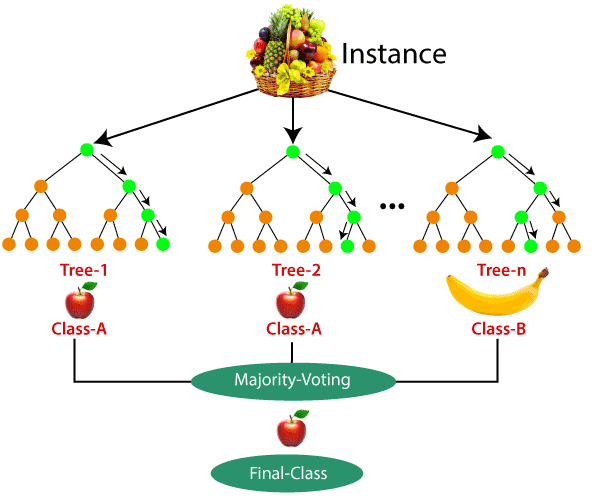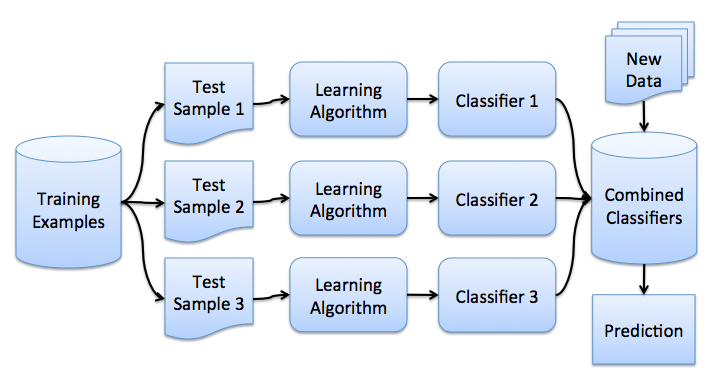# Understanding The Random Forest

## IntroductionSource: section.io

Random Forest is a supervised  Machine Learning Algorithm which is the extended version of the Decision Tree. The problem that arises in the Decision Tree is Overfitting. The algorithm tries to accurately fit the sample data according to the sample output and performs well on the sample data. But may perform very poorly in the case of the testing data. Random Forest says that let's not rely on only one Decision Tree. Instead, we will use multiple Decision Trees and select the majority result out of them. We will use the same training examples to build numerous Decision Trees, but they all will be different from each other. So, how is this possible? We will not use the same features to build the Decision Tree but will use some randomness to get to the point where one outlier might affect a few of the Decision Trees, but not all of the Decision Trees. Let's now talk about the formation of the Random Forest.

## Bagging

We are given some training data with columns as the features and rows as the data points or observations. We want to build a classifier that will provide us with the prediction. In Random Forest, we use multiple classifiers. The data fed in each of the classifiers should be different from each other. We use a method called BaggingBagging is the shortcut of the Bootstrap Aggregation Algorithm. Bagging says that if we have 'm' data points, let's select 'm' out of it, but with replacement, i.e., one data point can come multiple times. In this way, if any data point is present more than once, some of them must be missing.

Along with the Bagging, we make Feature Selection as well. In Feature selection, we are not going to train a Decision Tree based on all the features. If there are  'n' features, let's select 'k's out of it randomly. But with no replacement.  The very standard number to choose the feature is n out of n features. In this way, each of the Decision Trees is trained on different features and different training datasets. The good part about the feature selection is that if there is one feature that is creating a problem for us, we will have some trees that will not have that particular feature and hopefully will be able to reduce the overfitting problem.

Decision Trees and Random Forest can be used to solve regression problems (when we have to prodigy the value out of continuous range). For example, if we have to predict the stock price, the age of a person, etc., which is not a classification problem. We don't have a set of classes out of which we have to predict the value. We have a continuous range to predict any value in between.

Let's say we have a diabetes dataset and the prediction we are doing is the HbA1c (a test that measures the amount of blood sugar attached to the hemoglobin). Decision Trees that we have discussed are completely based on the classification. For regression prediction, we have to make some changes; the basic algorithm still stays the same. At any node, we will predict the mean as an output rather than the majority. We will divide a node by selecting a feature so that the mean squared error is minimum.

https://www.codingninjas.com/free-content/machine-learning-deep-learning-course/content/random-forests

• Preprocessing is not much required, missing values are handled.
• The Random Forest algorithm is Less prone to overfitting than the Decision Tree and other algorithms.
• The Random Forest algorithm Outputs importance of features which is very useful
• The Random Forest algorithm can be used as dimensionality reduction technique.
• The Random Forest algorithm has the capacity to handle large datasets with higher dimensionality.

• The Random Forest algorithms may change considerably by small changes in the data.
• The Random Forest algorithm calculation can go more complex than the other algorithms.
• The Random Forest algorithm requires higher time to train the model as a large number of trees are involved.
• The Random Forest  algorithm is not very suitable for regression analysis.
• The Random Forest algorithm is relatively less interpretable than the Decision Tree and other algorithms.

## FAQs

Q1) The Random Forest is good over Decision Tree because -
-> There is Overfitting in Decision Tree

Q2) Pick the correct option/s regarding using Decision Trees for regression-
a) Predicted value is the mean of all the samples that belong to that node.
b) Predicted value is the minimum of all the samples that belong to that node.
c) Split is based on the accuracy
d) Split is based on the MSE ( Mean Squared Error)
-> a) Predicted value is the mean of all the samples that belong to that node.
d) Split is based on the MSE ( Mean Squared Error)

Q3) What is Out-of-Bag Error?
-> In Random forests, there is no need for separate testing data to validate the result. As the forest is built on training data, each tree is tested on one-third of the samples that are not used in making that tree. This is known as the out-of-bag error, which is an internal error estimate of a Random forest as it is being constructed.

Q4) What are Bagging trees?
-> Bagging is the method for improving the performance by aggregating the result of weak learners.
In Bagging trees, individual trees are independent of each other.

Q5) What are Gradient boosting trees?
-> In Gradient boosting trees, we introduce a new regression tree to compensate for the shortcomings of the existing model.
We can use the Gradient Descent algorithm to minimize the loss function.

## Key Takeaways

So this is the end of the introduction to the Random Forest Algorithm. I hope you must have enjoyed the discussion. For further exploration of ML algorithms, you could visit Coding ninjas.

Thank you a lot, Happy Learning 😊😊